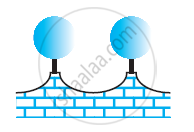# The Front Compound Wall of a House is Decorated by Wooden Spheres of Diameter 21 Cm, Find the Cost of Paint Required If Silver Paint Costs 25 Paise per Cm^2 and Black Paint Costs 5 Paise per Cm^2. - Mathematics

The front compound wall of a house is decorated by wooden spheres of diameter 21 cm, placed on small supports as shown in the given figure. Eight such spheres are used for this purpose, and are to be painted silver. Each support is a cylinder of radius 1.5 cm and height 7 cm and is to be painted black. Find the cost of paint required if silver paint costs 25 paise per cm2 and black paint costs 5 paise per cm2.#### Solution

Radius (r) of wooden sphere = (21/2)cm = 10.5cm

Surface area of wooden sphere = 4πr2

=[4xx22/7xx(10.5)^2]cm^2 = 1386cm^2

Radius (r1) of the circular end of cylindrical support = 1.5 cm

Height (h) of cylindrical support = 7 cm

CSA of cylindrical support = 2πrh

=[2xx22/7xx(1.5)xx7]cm^2 = 66cm^2

Area of the circular end of cylindrical support = πr2

=[22/7xx(1.5)^2]cm^2=7.07cm^2

Area to be painted silver = [8 × (1386 − 7.07)] cm2

= (8 × 1378.93) cm2 = 11031.44 cm2

Cost for painting with silver colour = Rs (11031.44 × 0.25) = Rs 2757.86

Area to be painted black = (8 × 66) cm= 528 cm2

Cost for painting with black colour = Rs (528 × 0.05) = Rs 26.40

Total cost in painting = Rs (2757.86 + 26.40)

= Rs 2784.26

Therefore, it will cost Rs 2784.26 in painting in such a way.

Concept: Volume of a Sphere
Is there an error in this question or solution?

#### APPEARS IN

RD Sharma Mathematics for Class 9
Chapter 21 Surface Areas and Volume of a Sphere
Exercise 21.1 | Q 13 | Page 9
NCERT Class 9 Maths
Chapter 13 Surface Area and Volumes
Exercise 13.9 | Q 2 | Page 237